### Vous n'êtes pas connecté !Vous devez être connecté pour activer des leçons et les ajouter à vos favoris.
Pour vous connecter, commandez des crédits ou un abonnement sur tutorom.fr :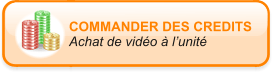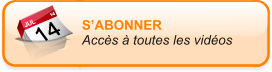Fermer cette fenêtre

### Activation de leçon(s)

Êtes-vous sur de vouloir utiliser un crédit ?

### Activation de leçon(s)

Êtes-vous sur de vouloir utiliser des crédits ?

### Activation de leçon(s)Vous n'avez pas assez de crédits disponibles pour débloquer cette(ces) leçon(s) !Fermer cette fenêtre

# Microsoft Excel 2013 Course

## Excel Basics » About Excel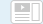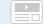• ###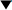Getting Started5 crédits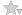(20:14)

• (02:54)
Open and Close a Workbook
• (03:23)
Move Around in a Workbook
• (04:34)
Save a Workbook
• (04:20)
Work with Accounts in Excel
• (01:43)
Create a New Workbook
• (03:20)
Use an Excel Template
• ###Entering Data7 crédits(31:18)

• (04:15)
• (04:10)
Enter Numbers
• (03:36)
Enter Dates
• (03:49)
AutoFill Dates
• (03:32)
AutoFill Numbers
• (03:56)
AutoFill Text
• (04:03)
Create an AutoFill Series
• (03:57)
• ###Rearranging a Worksheet4 crédits(18:38)

• (04:40)
Size Columns and Rows
• (03:45)
Insert & Delete Rows & Columns
• (04:18)
Copy or Move Data
• (03:31)
Copy or Move a Worksheet
• (02:24)
Transpose Data
• ###Basic Formulas & Functions11 crédits(46:52)

• (04:25)
Using SUM and AutoSum
• (04:24)
Doing Simple Math
• (03:20)
Order of Operations
• (03:54)
Calculating Averages
• (02:26)
Quick Analysis - Totals
• (03:08)
• (04:20)
Copy Formulas
• (04:00)
• (04:14)
Naming Ranges
• (04:11)
Calculating Using Names
• (04:17)
Understanding Errors
• (04:13)
Evaluating a Formula
• ###Formatting a Worksheet9 crédits(37:02)

• (04:48)
Format Numbers
• (02:25)
Format Dates
• (03:15)
Format with Color
• (03:38)
Use Worksheet Themes
• (04:28)
• (04:19)
Conditional Formatting pt. 1
• (04:23)
Conditional Formatting pt. 2
• (03:02)
Borrow Table Formats
• (04:21)
Create and Use Styles
• (02:23)
Paint and Clear Formats
• ###Creating Charts7 crédits(31:07)

• (04:43)
Create a Chart
• (03:21)
• (03:38)
Format a Chart pt. 1
• (04:19)
Format a Chart pt. 2
• (04:28)
Create a Pie Chart
• (03:23)
Understanding Chart Types
• (03:10)
Plot Data on Multiple Axes
• (04:05)
Create Sparklines
• ###Adding Art3 crédits(15:24)

• (03:53)
ClipArt and Photos
• (04:05)
Worksheet Backgrounds & Watermarks
• (03:10)
SmartArt
• (04:16)
Shapes
• ###More Complex Functions5 crédits(23:03)

• (04:03)
Using IF to Make Calculations
• (04:15)
Using SUMIF & COUNTIF
• (02:21)
Calculating with SUMPRODUCT
• (03:45)
Random Numbers with RANDBETWEEN
• (04:16)
Find and Use Functions
• (04:23)
Building a Mortgage Calculator
• ###Working with Tables5 crédits(20:46)

• (03:37)
Create and Format a Table
• (01:58)
Create a Chart of Table Data
• (03:26)
Calculations in a Table
• (03:59)
Use the Subtotal Function
• (04:22)
Filter Table Data
• (03:24)
Sort Data in a Table
• ###Working with PivotTables4 crédits(15:40)

• (02:50)
PivotTables Introduction
• (03:03)
Create a PivotTable
• (04:05)
Query a PivotTable
• (03:37)
Use Slicers
• (02:05)
Create a PivotChart
• ###Working with Large Worksheets5 crédits(24:21)

• (04:00)
Freeze Panes
• (04:06)
• (04:07)
Views
• (04:01)
Find & Replace
• (03:45)
Manipulate Data with Flash Fill
• (04:22)
Remove Duplicate Data
• ###Advanced Tools & Techniques11 crédits(46:13)

• (03:38)
Goal Seek
• (03:00)
Create and Use a Watch Window
• (04:01)
Create and Save a Template
• (03:20)
Calculate Across Multiple Worksheets
• (03:50)
• (04:12)
Use a Lookup Table pt. 1
• (04:26)
Use a Lookup Table pt. 2
• (04:47)
Import Data into Excel
• (02:39)
Find Precedents and Dependents
• (04:13)
Use Data Validation
• (03:30)
Enter Data Using Data Validation
• (04:37)
Work with Other Office Programs
• ###Printing a Worksheet5 crédits(20:32)

• (03:30)
Set Up a Worksheet for Printing
• (04:03)
Shrink to Fit
• (04:27)
Configure Printing Options
• (02:35)
Print a Chart
• (02:13)
Print a Selection
• (03:44)
Print to PDF
• ###Spreadsheet Design5 crédits(21:35)

• (04:15)
Design Basics
• (01:56)
Create a Data Area
• (04:03)
Work with Multi-Sheet Workbooks
• (03:49)
Protect a Worksheet
• (04:24)
Error Checking and Testing
• (03:08)
Spell Check and AutoCorrect
• ###Automating with Macros3 crédits(13:14)

• (02:24)
Understanding Macros
• (04:02)
Record a Macro
• (02:07)
Run a Macro
• (04:41)
Explore the Visual Basic Editor
• ###Working with Skydrive3 crédits(13:23)

• (03:22)
SkyDrive Introduction
• (04:17)
Save and Open SkyDrive Files
• (03:41)
Work in the Excel Web App
• (02:03)
How to Use SkyDrive
• ###Conclusion1 crédits(02:51)

• (02:51)
Course Wrap Up

• (03:00)